# JP2012008199A - Display control device, display system and display control method - Google Patents

## Info

Publication number
JP2012008199A
JP2012008199A JP2010141661A JP2010141661A JP2012008199A JP 2012008199 A JP2012008199 A JP 2012008199A JP 2010141661 A JP2010141661 A JP 2010141661A JP 2010141661 A JP2010141661 A JP 2010141661A JP 2012008199 A JP2012008199 A JP 2012008199A
Authority
JP
Japan
Prior art keywords
light source
control amount
image
input image
source control
Prior art date
Legal status (The legal status is an assumption and is not a legal conclusion. Google has not performed a legal analysis and makes no representation as to the accuracy of the status listed.)
Withdrawn
Application number
JP2010141661A
Other languages
Japanese (ja)
Inventor
Takumi Yoshimoto

Original Assignee
Fujitsu Ten Ltd

Priority date (The priority date is an assumption and is not a legal conclusion. Google has not performed a legal analysis and makes no representation as to the accuracy of the date listed.)
Filing date
Publication date
Application filed by Fujitsu Ten Ltd, 富士通テン株式会社 filed Critical Fujitsu Ten Ltd
Priority to JP2010141661A priority Critical patent/JP2012008199A/en
Publication of JP2012008199A publication Critical patent/JP2012008199A/en
Application status is Withdrawn legal-status Critical

• 238000009795 derivation Methods 0 abstract 4

## Images

•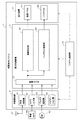•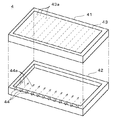••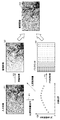•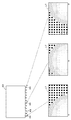•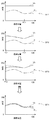••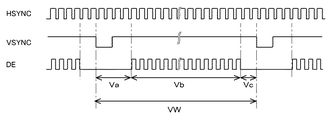•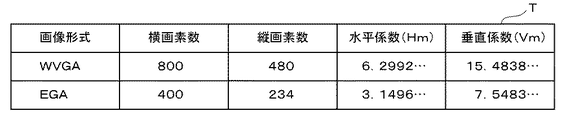•••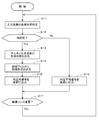•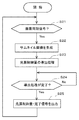•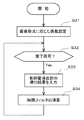•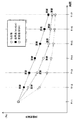•••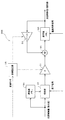•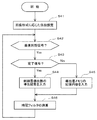•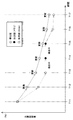•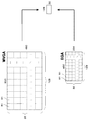•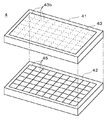## Abstract

The brightness of an image displayed on a display device and the amount of light of a backlight are prevented from greatly deviating from each other.
In a vehicle-mounted display system, a control amount deriving unit 345 derives a light source control amount based on the brightness of an input image displayed on a display device 4, and a time filter 346 uses the derived light source control amount in a time axis direction. Smoothing in The coefficient α, which is a level that reflects the light source control amount derived most recently in the processing result of the time filter 346, is set higher as the size of the input image is smaller. For this reason, even when the derivation time of the light source control amount (derived value) is relatively long due to the relatively small size of the input image, the light source control amount (used for adjusting the light amount of the light source of the backlight 42 ( The most recently derived light source control amount (derived value) is immediately reflected in the (used value). As a result, it is possible to prevent the brightness of the image displayed on the display device from greatly deviating from the amount of light of the backlight 42.
[Selection] Figure 3

## Description

The present invention relates to a technique for controlling a backlight that illuminates a screen of a display device.

A display device using a liquid crystal panel usually illuminates the screen from the back using a backlight. The display device realizes display of various contents by changing the transmittance of light from the backlight for each pixel. Such a backlight generally illuminates the entire screen of the liquid crystal panel with a uniform amount of light.

In contrast, a backlight is configured so that the screen of the liquid crystal panel can be illuminated with a plurality of light sources, and the light amount of the light source corresponding to each region is adjusted according to the brightness distribution in the image displayed on the screen. A control device has been proposed (see, for example, Patent Document 1). Specifically, the light quantity of the light source corresponding to the bright area in the image is made relatively large, and the light quantity of the light source corresponding to the dark area is made relatively small. If this technology is adopted, the power consumption can be effectively reduced as compared with the case where the entire screen is illuminated with a uniform amount of light.

JP-A-3-71111

When adjusting the light amount of the backlight according to the brightness of the image as described above, first, a light source control amount for defining the light amount of the light source is derived based on the input image. Next, in order to prevent flickering on the screen, the derived light source control amount is passed through a time filter, and the light source control amount is smoothed in the time axis direction. That is, the rapid change of the light source control amount is mitigated by the time filter. Then, the light amount of the light source of the backlight is adjusted based on the light source control amount that is the calculation result of the time filter.

In order to derive the light source control amount based on the input image, a plurality of calculations are required. The number of calculations required for deriving the light source control amount is not constant, and varies depending on the contents of the input image to be processed.

By the way, a configuration using a clock (CLK) indicating a display timing for each pixel of an input image can be considered as a reference for the timing of a plurality of operations for deriving a light source control amount. In such a configuration, the light source control amount derivation time varies depending on the size of the input image. This is because the number of clocks in a period of one frame of the input image (input image input cycle) varies depending on the size of the input image.

When a clock is used as a calculation timing reference for deriving the light source control amount, the number of clocks in a period of one frame corresponds to the number of times that calculations can be executed during synchronization. Therefore, the smaller the size of the input image, the smaller the number of clocks in the period for one frame, so the number of operations that can be performed during synchronization is reduced. The period of one frame (input image input cycle) corresponds to the reciprocal of the frame rate (refresh rate) (for example, 60 Hz), and is usually constant (for example, 1/60 seconds) depending on the display device. Accordingly, the smaller the size of the input image, the higher the possibility that the derivation time of the light source control amount will not be completed within one frame period and the light source control amount derivation time will be longer.

In this way, when the light source control amount derivation time becomes long, the time filter cannot be calculated during that time. For this reason, during the derivation of the light source control amount, the light source control amount that is the latest calculation result of the time filter is continuously used for adjusting the light amount of the light source of the backlight. Further, even after the derivation of the light source control amount is completed, the calculation result of the time filter is affected by the past light source control amount derived several frames before. As a result, during or after the derivation of the light source control amount, the brightness of the image actually displayed on the display device and the amount of light of the backlight may greatly deviate, which may cause an unnatural display.

The present invention has been made in view of the above problems, and the brightness of the image displayed on the display device and the amount of light of the backlight even when the control amount derivation time is prolonged due to the size of the input image. It is an object of the present invention to provide a display control device that can prevent a significant deviation from the above.

In order to solve the above-mentioned problem, the invention of claim 1 is a display control device that controls a backlight that illuminates a screen of a display device with at least one light source, and the brightness of an input image displayed on the display device is controlled. On the basis of derivation means for deriving a control amount for defining the light amount of the light source of the backlight by repeating the calculation for each clock corresponding to one pixel of the input image, and the control amount derived to the derivation means, Smoothing means for performing smoothing processing for smoothing in the time axis direction, adjustment means for adjusting the light amount of the light source based on a processing result by the smoothing means, and determination means for determining the size of the input image And a process changing means for changing the degree of reflecting the control amount derived most recently in the processing result of the smoothing means according to the size of the input image. .

In the display control apparatus according to claim 1, the processing change unit is configured such that the processing change unit is a control amount that is most recently derived from the processing result of the smoothing unit as the size of the input image is smaller. Increase the degree of reflection.

The display control apparatus according to claim 1 or 2, wherein the smoothing means executes the smoothing process at an input cycle of the input image, and the smoothing process is performed at the time point when the smoothing process is performed. When the derivation of the control amount by the derivation unit is not completed, the smoothing process is executed using the control amount derived in the past by the derivation unit.

According to a fourth aspect of the present invention, there is provided a display system comprising: the display control device according to any one of the first to third aspects; and a display device that displays an image output from the display control device. Yes.

The invention of claim 5 is a display control method for controlling a backlight that illuminates a screen of a display device with at least one light source, and (a) based on the brightness of an input image displayed on the display device. A step of deriving a control amount for defining the light amount of the light source of the backlight by repeating the calculation for each clock corresponding to one pixel of the input image; and (b) the control amount derived in the step (a). Performing a smoothing process for smoothing in the time axis direction, (c) adjusting the light amount of the light source based on the processing result of the (b) process, and (d) the input image A step of determining a size, and (e) a step of changing a degree of reflecting the control amount most recently derived in the processing result of the step (b) according to the size of the input image. .

According to the first to fifth aspects of the present invention, the extent to which the control amount derived most recently is reflected in the processing result of the smoothing means is changed according to the size of the input image. For this reason, even when the derivation time of the control amount becomes long due to the size of the input image, the derived control amount can be reflected in actual control promptly. As a result, it is possible to prevent the brightness of the image displayed on the display device from greatly deviating from the amount of light of the backlight.

In particular, according to the invention of claim 2, even when the size of the input image is relatively small, the number of operations per input cycle of the input image is reduced, and the control amount derivation time is increased. The derived control amount can be quickly reflected in actual control.

In particular, according to the invention of claim 3, when the derivation of the control amount is not completed at the time of execution of the smoothing process, the smoothing process is executed based on the control amount that has been derived in the past, and the process The light quantity of the light source is adjusted based on the result. For this reason, the light quantity of the light source can be adjusted with an appropriate control amount even during the derivation of the control amount.

FIG. 1 is a block diagram showing a configuration of an in-vehicle display system. FIG. 2 is an exploded perspective view illustrating an example of the configuration of the display device. FIG. 3 is a diagram illustrating a functional configuration of the image correction unit and the backlight control unit. FIG. 4 is a diagram for explaining the outline of the power reduction process. FIG. 5 is a diagram showing luminance distribution data. FIG. 6 is a diagram illustrating an example of processing for adjusting the light source control amount. FIG. 7 is a diagram illustrating the relationship between the horizontal synchronization signal and other control signals. FIG. 8 is a diagram illustrating the relationship between the vertical synchronization signal and other control signals. FIG. 9 is a diagram illustrating an example of the determination table. FIG. 10 is a diagram illustrating an example of processing for generating a thumbnail image. FIG. 11 is a diagram illustrating a configuration of the time filter according to the first embodiment. FIG. 12 is a diagram illustrating a flow of processing relating to determination of the image format of the input image. FIG. 13 is a diagram illustrating a flow of processing related to the derivation of the light source control amount. FIG. 14 is a diagram illustrating a flow of processing of the time filter according to the first embodiment. FIG. 15 is a time chart illustrating an example of a change in the light source control amount. FIG. 16 is a time chart illustrating an example of a change in the light source control amount. FIG. 17 is a time chart illustrating an example of a change in the light source control amount. FIG. 18 is a diagram illustrating a configuration of a time filter according to the second embodiment. FIG. 19 is a diagram illustrating a flow of processing of the time filter according to the second embodiment. FIG. 20 is a time chart illustrating an example of a change in the light source control amount. FIG. 21 is a diagram illustrating another example of processing for generating a thumbnail image. FIG. 22 is a diagram illustrating another example of the configuration of the display device.

Hereinafter, embodiments of the present invention will be described with reference to the drawings.

<1. First Embodiment>
<1-1. Configuration>
FIG. 1 is a block diagram showing a configuration of an in-vehicle display system 1 according to the present embodiment. The in-vehicle display system 1 is configured as, for example, a navigation system for a vehicle such as an automobile, and has a function of being mounted on the vehicle and displaying various images to users in the vehicle interior. For example, the in-vehicle display system 1 includes a map image for navigation guidance, an image based on a television broadcast signal received by the antenna 91, a vehicle periphery image showing the periphery of the vehicle photographed by an in-vehicle camera 92 provided in the vehicle, In addition, an image obtained by reading a video disc 93 such as a DVD can be displayed.

As shown in FIG. 1, the in-vehicle display system 1 provides a display device 4 capable of various displays, a display control device 3 that controls the display of the display device 4, and a video source for display on the display device 4. And an image providing unit 2 for performing the operation.

Furthermore, the in-vehicle display system 1 includes a system control unit 10 that controls the entire system. The system control unit 10 is configured by a microcomputer including a CPU, a RAM, a ROM, and the like. Various control functions for controlling the entire system are realized by the CPU of the system control unit 10 performing arithmetic processing according to a predetermined program. The system control unit 10 comprehensively controls operations of the video providing unit 2, the display control device 3, and the display device 4.

The display device 4 includes a liquid crystal panel 41 that displays an image and a backlight 42 that illuminates the screen of the liquid crystal panel 41. The in-vehicle display system 1 is installed on an instrument panel or the like of the vehicle so that the screen of the liquid crystal panel 41 of the display device 4 can be visually recognized by a vehicle occupant who is a user.

FIG. 2 is an exploded perspective view showing an outline of the configuration of the display device 4. The screen 43 of the liquid crystal panel 41 is composed of, for example, a plurality of pixels arranged two-dimensionally in the vertical and horizontal directions. The backlight 42 illuminates the screen 43 of the liquid crystal panel 41 from the back side. The liquid crystal panel 41 realizes display of various contents by changing the transmittance of light from the backlight 42 for each pixel.

The backlight 42 includes a plurality (for example, twelve in the present embodiment) of light sources 44 configured by LEDs (Light Emitting Diodes) or the like. The plurality of light sources 44 are arranged in a line in the vicinity of the position corresponding to the lower side of the screen 43 along the horizontal direction of the screen 43. The optical axis 44 a of each light source 44 extends along the vertical direction of the screen 43, and each light source 44 emits light toward a position corresponding to the upper side of the screen 43. Further, the screen 43 of the liquid crystal panel 41 is divided into a plurality of divided regions 43 a as many as the light sources 44 in the horizontal direction. The plurality of light sources 44 respectively correspond to the plurality of divided regions 43a. In other words, it can be said that the plurality of light sources 44 respectively correspond to a plurality of regions obtained by dividing the image displayed on the screen 43 in the horizontal direction. Each light source 44 is mainly responsible for the illumination of the corresponding segmented area 43 a of the screen 43 of the liquid crystal panel 41.

The backlight 42 is provided with a driver (not shown) corresponding to each light source 44. The driver can change the amount of light emitted from the corresponding light source 44. Therefore, the plurality of light sources 44 can emit light with independent light amounts. The driver receives a PWM (Pulse Width Modulation) signal, and changes the light amount of the corresponding light source 44 according to the duty ratio indicated by the PWM signal. That is, the higher the duty ratio, the larger the light amount, and the lower the duty ratio, the smaller the light amount. Therefore, the backlight 42 can illuminate the screen 43 of the liquid crystal panel 41 with different amounts of light depending on the region.

Returning to FIG. 1, the video providing unit 2 includes a broadcast receiving unit 21, a camera input unit 22, a disk reading unit 23, and a navigation unit 24. Images output from the units 21, 22, 23, and 24 of the video providing unit 2 are input to the display control device 3 as input images together with various control signals such as synchronization signals. One input image is input to the display control device 3 for each vertical synchronization signal. For this reason, the input image is input to the display control device 3 in a predetermined input cycle continuously in time. The input cycle of this input image corresponds to the reciprocal of the frame rate (refresh rate) of the display device 4. In the present embodiment, for example, the frame rate of the display device 4 is 60 Hz, and the input cycle of the input image is 1/60 seconds.

The size of the input image can be represented by an image format indicating the number of vertical and horizontal pixels of the image such as WVGA or EGA. The image format of the input image input from the video providing unit 2 is normally adjusted in the video providing unit 2 to match the screen resolution indicating the number of vertical and horizontal pixels of the screen 43 of the display device 4. For example, when the screen resolution of the display device 4 is WVGA (800 horizontal by 480 vertical pixels), the image format of the input image is also WVGA. When the screen resolution of the display device 4 is EGA (horizontal 400 × vertical 234 pixels), the image format of the input image is also EGA.

The input image is subjected to predetermined processing in the display control device 3 and then continuously output from the display control device 3 together with various control signals at a predetermined cycle (for example, 1/60 seconds). Thereby, in the display device 4, a plurality of temporally continuous images are displayed as moving images.

The broadcast receiving unit 21 decodes a broadcast signal such as a television broadcast or a data broadcast received by the antenna 91 mounted on the vehicle, acquires an image indicating the broadcast content, and outputs the image to the display control device 3.

The camera input unit 22 is connected to the in-vehicle camera 92, acquires an image showing the periphery of the vehicle photographed by the in-vehicle camera 92, and outputs the acquired image to the display control device 3.

The disc reading unit 23 is configured as a reading device for a video disc 93 such as a DVD, acquires an image indicating the recorded content of the video disc 93 and outputs it to the display control device 3.

Moreover, the navigation part 24 is comprised as an electronic board which integrated the function for navigation guidance. The navigation unit 24 outputs a map image for navigation guidance and the like to the display control device 3.

The display control device 3 is configured as, for example, an ASIC (Application Specific Integrated Circuit), and has a function of processing an input image to be displayed and a function of controlling the operation of the backlight 42.

As illustrated in FIG. 1, the display control device 3 includes an image input unit 31, an image correction unit 33, and a backlight control unit 34 as functional units that realize predetermined functions.

The image input unit 31 inputs an input image as a video source from each unit 21, 22, 23, 24 of the video providing unit 2. The image input unit 31 performs switching based on an instruction from the system control unit 10, selects any one of four types of video sources input from the video providing unit 2 as a target to be displayed, and performs image correction. To the unit 33 and the backlight control unit 34.

The image correction unit 33 amplifies the input image to generate an amplified image, and outputs the amplified image to the liquid crystal panel 41 of the display device 4 for display. Further, the backlight control unit 34 adjusts the light amounts of the plurality of light sources 44 of the backlight 42 of the display device 4 according to the brightness of the input image.

FIG. 3 is a diagram illustrating the functional configuration of the image correction unit 33 and the backlight control unit 34 in more detail. As shown in the figure, the image correction unit 33 includes an image amplification unit 331 and an image output unit 332. The backlight control unit 34 includes an image format determination unit 341, a determination memory 342, a thumbnail generation unit 343, a control amount derivation unit 345, a time filter 346, and a duty control unit 348. Details of these functions will be described below.

<1-2. Power reduction processing>
The display control device 3 can perform a power reduction process for reducing the power consumption of the display device 4. In the configuration shown in FIG. 3, the control amount deriving unit 345 of the backlight control unit 34 performs processing for suppressing the amount of light of the backlight 42, and the image amplification unit 331 of the image correction unit 33 amplifies the luminance of the input image. Perform the process. The power reduction process is mainly realized by the processes of the control amount deriving unit 345 and the image amplifying unit 331. FIG. 4 is a diagram for explaining the outline of the power reduction process.

The control amount deriving unit 345 derives a plurality of light source control amounts that respectively define the light amounts of the plurality of light sources 44 of the backlight 42 (processing P1). In order to derive one light source control amount for one light source 44, the control amount deriving unit 345 derives the same number of light source control amounts as the number of light sources 44 (for example, 12 in this embodiment). This light source control amount indicates the amount of light to be emitted by the light source 44 as a ratio (%) to the maximum amount of light.

The control amount deriving unit 345 derives the light source control amount of the light source 44 based on the luminance distribution indicating the brightness of the input image G1. Specifically, in the input image G1, the light source control amount of the light source 44 corresponding to the region with relatively high luminance is set to be relatively large, and conversely, the light source control amount of the light source 44 corresponding to the region with relatively low luminance. Is set relatively small. A specific method for deriving the light source control amount will be described later.

The light source control amount derived in this way is subjected to predetermined processing by the time filter 346 and then input to the duty control unit 348 and the image amplification unit 331 of the image correction unit 33.

The light source control amount input to the duty control unit 348 is used as the duty ratio of the PWM signal. The duty control unit 348 transmits the PWM signal in which the duty ratio is set to the driver of each light source 44 of the backlight 42 (processing P2).

Thereby, in the backlight 42, the light quantity of the some light source 44 is adjusted separately. That is, the light amount of the light source 44 corresponding to the region where the luminance of the input image G1 is high is suppressed to be small, and the light amount is relatively large. Conversely, the light quantity of the light source 44 corresponding to the area where the luminance of the input image G1 is low is greatly suppressed, and the light quantity is relatively small. Thus, by suppressing the light quantity of the backlight 42, power consumption is reduced compared with the case where all the light sources 44 of the backlight 42 emit light uniformly with the maximum light quantity (100% light quantity). In FIG. 4, in the backlight 42, dark hatching is used for a portion with a relatively small amount of light, and thin hatching is used for a portion with a relatively large amount of light.

On the other hand, the pixel value of the input image G1 is amplified in order to reduce the visual effect when the light amount of the backlight 42 is suppressed. The image amplifying unit 331 of the image correcting unit 33 amplifies the pixel value of the input image G1 according to the input light source control amount, and generates an amplified image G2 (processing P3).

Specifically, the light quantity of the backlight 42 when the input image G1 on the screen 43 is illuminated is derived for each pixel position of the input image G1 based on the light source control amounts of the plurality of light sources 44. The amplification factor is set to be relatively large for the pixel at a position where the light amount of the backlight 42 is relatively small. On the contrary, the amplification factor is set to be relatively small for a pixel at a position where the light amount of the backlight 42 is relatively large. Then, based on the set amplification factor, the pixel value (RGB value or luminance value) of each pixel of the input image G1 is amplified, and an amplified image G2 is generated. The generated amplified image G2 is output from the image output unit 332 and displayed on the liquid crystal panel 41.

By adjusting the light amount of the backlight 42 and displaying the amplified image G2 on the liquid crystal panel 41 at the same time, the observation image G3 observed by the user on the screen 43 of the liquid crystal panel 41 becomes natural. . This is because the influence on the visibility due to the suppression of the light amount of the backlight 42 is offset by the amplification of the pixel value of the image. As a result, the power consumption can be effectively reduced while making the observation image G3 natural.

<1-3. Derivation of light source control amount>
Next, a method in which the control amount deriving unit 345 derives the light source control amount of each of the plurality of light sources 44 based on the luminance distribution of the input image (processing P1 in FIG. 4) will be described. The control amount deriving unit 345 includes a memory that stores data indicating the characteristics of each light source 44, and derives a light source control amount using this data.

As shown in FIG. 5, the memory of the control amount deriving unit 345 has a screen when only one light source 44 emits light with the maximum light amount in a state where an image of one white color is displayed on the screen 43 of the liquid crystal panel 41. The luminance distribution data LT indicating the luminance distribution at 43 is stored. The luminance distribution data LT includes a plurality of luminance values corresponding to the position of each pixel on the screen 43. Such luminance distribution data LT is prepared for each light source 44 and stored in the memory.

The luminance distribution data LT shown on the left side in FIG. 5 indicates the luminance distribution when only the light source 44 arranged at the left end is caused to emit light. Further, the luminance distribution data LT shown at the center indicates the luminance distribution when only the light source 44 arranged at the substantially central portion emits light. Further, the luminance distribution data LT shown on the right side shows the luminance distribution when only the light source 44 arranged at the right end is caused to emit light. In the figure, dark hatching is used for portions with relatively low luminance, and thin hatching is used for portions with relatively high luminance. As shown in the figure, the brightness decreases as the distance from the light source 44 that emits light increases.

Each light source 44 does not emit light alone, but actually, each of the plurality of light sources 44 emits light with a light amount defined by the light source control amount, and these lights are combined at each position on the screen 43. . For this reason, the luminance (hereinafter referred to as “backlight luminance”) LL at the position of each pixel of the screen 43 by the actual illumination of the backlight 42 can be expressed by the following equation (1).

In Equation 1, n is a number identifying the light source 44 (n = 1 to 12 in the present embodiment), M is the number of light sources 44 (M = 12 in the present embodiment), and LD (n) is nth. The luminance value at the position of the pixel indicated by the luminance distribution data LT of the light source 44 and RATE (n) indicate the light source control amount of the nth light source 44, respectively. The control amount deriving unit 345 compares the backlight luminance LL expressed by Equation 1 with the luminance of the input image (hereinafter referred to as “image luminance”) at the same pixel position, so that A light source control amount (RATE (n)) is determined.

In calculating the light source control amount, the control amount deriving unit 345 sets a processing target for each horizontal line included in the input image. Then, the image luminance in the horizontal line to be processed is compared with the backlight luminance corresponding to the position, and the light source control amount is adjusted so that the image luminance and the backlight luminance substantially coincide.

FIG. 6 is a diagram for explaining an example of processing for adjusting the light source control amount with respect to one horizontal line as a processing target. In the figure, the horizontal axis indicates the pixel position on the horizontal line to be processed, and the vertical axis indicates the luminance.

First, in the horizontal line, the pixel position where the difference between the image luminance PL and the backlight luminance LL is the largest is determined. In the state ST1 shown in the figure, the difference between the image brightness PL and the backlight brightness LL is the largest at the position Px1. For this reason, the light source control amount of the light source 44 corresponding to the position Px1 is reduced by a predetermined value (for example, 5 (%)). As a result, the backlight luminance LL decreases in the vicinity of the position Px1 (state ST2).

Next, in the state ST2, the difference between the image brightness PL and the backlight brightness LL becomes the largest at the position Px2. For this reason, the light source control amount of the light source 44 corresponding to the position Px2 is decreased by a predetermined value, and the backlight luminance LL is decreased in the vicinity of the position Px2 (state ST3).

Similarly, the position of the pixel where the difference between the image brightness PL and the backlight brightness LL is maximized is determined, and the light source control amount of the light source 44 corresponding to this position is decreased by a predetermined value (for example, 5 (%)). Is done. Such calculation is repeated, and finally, the image brightness PL and the backlight brightness LL substantially coincide with each other in the horizontal line of interest (state ST4).

In the example of the process illustrated in FIG. 6, the example in which the initial value of the backlight luminance LL is greater than the image luminance PL has been described. On the other hand, when the initial value of the backlight luminance LL is smaller than the image luminance PL, the light source control amount of the light source 44 corresponding to the position where the difference between the image luminance PL and the backlight luminance LL is the largest is a predetermined value. Will be increased.

The control amount deriving unit 345 executes such processing for each horizontal line while changing the horizontal line to be processed from the lowest level to the highest level of the horizontal line included in the input image. When changing the horizontal line to be processed, the light source control amount adjusted in the previous horizontal line is used as an initial value in the processing of the next horizontal line. Then, the light source control amount when the processing relating to the uppermost stage of the horizontal line included in the input image is completed is set as the light source control amount used for the control.

Thus, when the control amount deriving unit 345 derives the light source control amount, an operation for increasing or decreasing the light source control amount corresponding to the pixel position having the maximum luminance difference (hereinafter referred to as “increase / decrease operation”) is repeatedly executed. To do. As a reference for the timing of executing this increase / decrease calculation, a clock (CLK) indicating the display timing for each pixel of the input image is used.

The number of increase / decrease operations required until the control amount derivation unit 345 completes the derivation of the light source control amount based on one input image differs depending on the content of the target input image (the luminance distribution state). . Therefore, the time until the derivation of the light source control amount based on one input image is different every time. When the light source control amount derivation process is completed, the control amount derivation unit 345 outputs a completion signal indicating that the derivation process is completed together with the derived light source control amount to the time filter 346 in accordance with the timing of the vertical synchronization signal. (See FIG. 3).

<1-4. Generation of thumbnail images>
As described above, when deriving the light source control amount by the control amount deriving unit 345, the image format of the input image is taken into account because there is an operation for comparing the image luminance and the backlight luminance at the same pixel position. Processing is required.

In the display control apparatus 3 of the present embodiment, a thumbnail image with a fixed number of vertical and horizontal pixels is generated from the input image in order to appropriately derive the light source control amount from the input images of various assumed image formats. The light source control amount is derived using the generated thumbnail image. The number of vertical and horizontal pixels of the generated thumbnail image is smaller than the number of vertical and horizontal pixels of the input image (that is, the number of vertical and horizontal pixels of the screen 43 of the display device 4), and is constant regardless of the image format of the input image. That is, whether the input image is WVGA or EGA, a thumbnail image having a constant size smaller than that is generated. Then, the light source control amount is derived by a derivation process suitable for the size of the thumbnail image. The brightness distribution data LT is prepared in advance according to the size of the thumbnail image. As a result, the display control device 3 of the present embodiment can appropriately derive the light source control amount regardless of the image format of the input image.

Such thumbnail image generation processing is mainly realized by the image format determination unit 341 and the thumbnail generation unit 343 (see FIG. 3). The image format determination unit 341 determines the image format of the input image (that is, the size of the input image), and inputs the determination result to the thumbnail generation unit 343. The thumbnail generation unit 343 generates a thumbnail image with a fixed number of vertical and horizontal pixels from the input image based on the determination result of the image format. In the present embodiment, for example, a thumbnail image of horizontal 128 × vertical 32 pixels is generated.

The image format determination unit 341 uses a control signal input to the display control device 3 together with the input image in order to determine the image format of the input image. The control signal is input from the image input unit 31 to the image format determination unit 341. The control signal includes a vertical synchronization signal (VSYNC), a horizontal synchronization signal (HSYNC), a data enable signal (DE), a clock (CLK), and the like. When determining the image format, the image format determination unit 341 first derives the number of horizontal pixels and the number of vertical pixels of the input image based on the control signal.

FIG. 7 is a diagram illustrating the relationship between the horizontal synchronization signal (HSYNC) and other control signals. The horizontal synchronization signal (HSYNC) is a signal that becomes “L” in a predetermined cycle, and indicates the input timing of one horizontal line of the input image. As shown in the figure, the horizontal synchronization interval HW, which is a cycle in which the horizontal synchronization signal (HSYNC) becomes “L”, is a blank period Ha before displaying an image, a display period Hb for displaying an image, and after displaying an image. The blank period Hc is a combined period. One horizontal line is displayed during the display period Hb.

In the display period Hb, the data enable signal (DE) becomes “H”, and the data enable signal (DE) becomes “L” in the other blank periods Ha and Hc. Further, as described above, the clock (CLK) is a periodic pulse signal indicating the timing for displaying one pixel. For this reason, the display period Hb is detected based on the data enable signal (DE), and the number of pixels (pulse number) included in the display period Hb is counted to determine the number of pixels included in one horizontal line, that is, The number of horizontal pixels of the input image can be obtained. The image format determination unit 341 derives the number of horizontal pixels of the input image based on such a principle. As shown in FIG. 7, the clock (CLK) is a signal that continues even during the blank period (the period in which the data enable signal (DE) is “L”).

FIG. 8 is a diagram illustrating the relationship between the vertical synchronization signal (VSYNC) and other control signals. The vertical synchronization signal (VSYNC) is a signal that becomes “L” in a predetermined cycle, and indicates the input timing of one input image. As shown in the figure, the vertical synchronization interval VW, which is a cycle in which the vertical synchronization signal (VSYNC) is “L”, is a blank period Va before displaying an image, a display period Vb displaying an image, and after displaying an image. The blank period Vc is a combined period. An entire input image is displayed during the display period Vb.

In the display period Vb, the data enable signal (DE) is repeatedly set to “H”, and in the other blank periods Va and Vc, the data enable signal (DE) is continuously set to “L”. The number that the data enable signal (DE) becomes “H” in one cycle of the vertical synchronization signal (VSYNC) matches the number of horizontal lines. Therefore, the number of horizontal lines, that is, the number of vertical pixels of the input image can be obtained by counting the number of times the data enable signal (DE) is “H” in one cycle of the vertical synchronization signal (VSYNC). The image format determination unit 341 derives the number of vertical pixels of the input image based on such a principle.

When the image format determination unit 341 derives the number of horizontal pixels and the number of vertical pixels of the input image in this way, the image format is determined based on the number of horizontal pixels and the number of vertical pixels. In this determination, the determination table stored in the determination memory 342 is referred to. This determination table can be rewritten by transmitting a predetermined signal to the determination memory 342.

FIG. 9 is a diagram illustrating an example of the determination table T. As illustrated in FIG. This determination table T shows image formats that can be determined by the image format determination unit 341, and a plurality of image formats are registered. In the example of the figure, two image formats of WVGA (horizontal 800 × vertical 480 pixels) and EGA (horizontal 400 × vertical 234 pixels) are registered in the determination table T. In the determination table T, values of “horizontal pixel number”, “vertical pixel number”, “horizontal coefficient”, and “vertical coefficient” are associated with each image format. Of these values, the “horizontal coefficient” and the “vertical coefficient” are generation coefficients used for generating thumbnail images.

The image format determination unit 341 compares the derived combination of the number of horizontal pixels and the number of vertical pixels of the input image with the combination of the number of horizontal pixels and the number of vertical pixels of the image format registered in the determination table T. The image format of the input image can be determined. However, when the derived combination of the number of horizontal pixels and the number of vertical pixels of the input image does not correspond to any of the image formats registered in the determination table T, the image format determination unit 341 cannot determine the image format. The image format determination unit 341 outputs a determination possible signal when the image format can be determined, and outputs a determination impossible signal to each unit of the display control device 3 when the image format cannot be determined.

If the image format can be determined, the image format determination unit 341 acquires the horizontal coefficient and the vertical coefficient of the determined image format from the determination table T, and outputs them to the thumbnail generation unit 343 (see FIG. 3). . At the same time, the image format determination unit 341 outputs the determined image format to the time filter 346 (see FIG. 3).

The thumbnail generation unit 343 generates a thumbnail image of a certain size from the input image based on the generation coefficient (horizontal coefficient and vertical coefficient) input in this way.

FIG. 10 is a diagram showing an outline of processing for generating the thumbnail image. The thumbnail generation unit 343 generates a thumbnail image of a certain size by thinning out pixels of the input image in both the horizontal direction and the vertical direction.

With respect to the horizontal direction of the image, the pixel row of the input image is thinned out based on the input horizontal coefficient (hereinafter referred to as symbol Hm). Here, the horizontal coefficient Hm indicates the interval between pixel columns left by thinning, and is a value derived from the following equation 2.

In Equation 2, HPn is the number of horizontal pixels of the input image, and HPs is the number of horizontal pixels of the thumbnail image. In the present embodiment, the number of horizontal pixels HPs of the thumbnail image is 128. Therefore, for example, when the input image is WVGA (HPn = 800), the horizontal coefficient Hm = 6.29292..., And when the input image is EGA (HPn = 400), the horizontal coefficient Hm = 3.1496. In the input image, the horizontal coefficient Hm is multiplied by a natural number and rounded off, leaving the pixel array arranged at the position of the value and discarding the other pixel arrays, so that the number of horizontal pixels of the image can be set to 128. .

As for the vertical direction of the image, the horizontal line of the input image is thinned out based on the input vertical coefficient (hereinafter, the symbol Vm is used). Here, the vertical coefficient Vm indicates the interval between horizontal lines left by thinning, and is a value derived from the following equation (3).

In Equation 3, VPn is the number of vertical pixels of the input image, and VPs is the number of vertical pixels of the thumbnail image. In the present embodiment, the number of vertical pixels VPs of the thumbnail image is 32. Therefore, for example, when the input image is WVGA (VPn = 480), the vertical coefficient Vm = 15.4838..., And when the input image is EGA (VPn = 234), the vertical coefficient Vm = 7.5483. Then, in the input image, the number of vertical pixels of the image is set to 32 by leaving the horizontal lines arranged at the position where the vertical coefficient Vm is multiplied by a natural number and rounded off, and discarding the other horizontal lines. it can.

Through such processing, the thumbnail generation unit 343 can generate a thumbnail image having a fixed size of 128 × 32 pixels regardless of the image format of the input image. The generated thumbnail image is used for a light source control amount derivation process by the control amount derivation unit 345.

<1-5. Configuration of time filter>
Next, the time filter 346 (see FIG. 3) will be described. The time filter 346 performs an operation of smoothing the light source control amount repeatedly derived to the control amount deriving unit 345 in the time axis direction.

The light source control amount derived by the control amount deriving unit 345 is in accordance with the brightness of the input image. For this reason, when there is a sudden change in the brightness of the input image, the light source control amount derived by the control amount deriving unit 345 also changes abruptly. If the light source control amount derived by the control amount deriving unit 345 is used for light amount adjustment as it is, it will feel that the light amount of the backlight changes rapidly and the screen flickers.

Therefore, in the in-vehicle display system 1, the light source control amount derived by the control amount deriving unit 345 is passed through the time filter 346, and the light source control amount that is the calculation result of the time filter 346 is used as the duty control unit 348 and the image amplification unit 331. And used for actual control (adjustment of light quantity and generation of an amplified image). The time filter 346 relaxes the change in the light source control amount by smoothing the light source control amount in the time axis direction. As a result, a sudden change in the light source control amount is alleviated, and flickering of the screen can be prevented. Hereinafter, the light source control amount derived to the control amount deriving unit 345 before passing through the time filter 346 is also referred to as “light source control amount (derived value)”, and the light source control amount used for actual control, which is the calculation result of the time filter 346. Is also referred to as “light source control amount (use value)”.

FIG. 11 is a diagram illustrating the configuration of the time filter 346. The number of time filters 346 having the configuration shown in FIG. 11 is prepared as many as the number of light sources 44 (that is, as many as the number of light source control amounts derived by the control amount deriving unit 345). Each time filter 346 corresponds to one light source 44, and based on the light source control amount (derived value) of the corresponding light source 44, the light source control amount (use value) of the corresponding light source 44 is obtained by calculation.

As shown in the figure, the time filter 346 includes two amplifiers 51 and 54, an adder 52, a use value memory 53, and a coefficient setting unit 55. The use value memory 53 receives a completion signal from the control amount deriving unit 345 (see FIG. 3), and the coefficient setting unit 55 receives the image format of the input image from the image format determining unit 341 (see FIG. 3). The

The amplifier 51 receives the light source control amount (derived value) most recently derived by the control amount deriving unit 345. In the amplifier 51, the light source control amount (derived value) is multiplied by a coefficient α (α <1), and the result is output to the adder 52.

The use value memory 53 is a memory for storing a light source control amount (use value). The light source control amount (use value) stored in the use value memory 53 is input to the amplifier 54. In the amplifier 54, the light source control amount (use value) is multiplied by (1−α), and the result is output to the adder 52.

The multiplication results of the two amplifiers 51 and 54 are added by the adder 52. As a result, Z n represented by the following equation 4 is output from the adder.

In Equation 4, In is a light source control amount (derived value) input to the amplifier 51, and Z n-1 is a light source control amount (use value) stored in the use value memory 53 before calculation. Derived Z n will be newly stored in the use-value memory 53. Z n stored in the use-value memory 53 is used to actual control (generation of light amount adjustment and amplification image) as the light source control amount (use value). At the same time, Z n stored in the use value memory 53 is also used as Z n−1 in the following equation 4.

The time filter 346 smoothes a plurality of light source control amounts (derived values) derived continuously in time in the time axis direction by repeating the calculation shown in Equation 4. The calculation of the time filter 346 shown in Formula 4 is executed every time a completion signal is input to the use value memory 53.

The coefficient α is set by the coefficient setting unit 55. As shown in Equation 4, the coefficient alpha, the Z n is the processing result of the time filter 346, and indicates the extent to reflect the light source control amount derived most recently (derived value) an In. Therefore, the higher the coefficient α, the more the light source control amount (use value) is affected by the light source control amount (derived value) derived most recently. That is, the higher the coefficient α, the more sensitively the light source control amount (use value) changes in accordance with the change in the light source control amount (derived value). The coefficient setting unit 55 sets the coefficient α according to the image format of the input image input from the image format determination unit 341.

<1-6. Relationship between image format and light source control amount derivation time>
As described above, the clock (CLK) indicating the display timing for each pixel of the input image is used as the reference of the increase / decrease calculation timing at which the control amount deriving unit 345 derives the light source control amount (derived value). When the clock (CLK) is used in this way, the light source control amount derivation time varies depending on the size of the input image, that is, the image format of the input image such as WVGA or EGA.

The number of clocks (CLK) in the period of one frame of the input image (= input image input period = vertical synchronization interval VW (see FIG. 8)) (hereinafter referred to as “total number of clocks”) is the size of the input image. It depends on. The smaller the size of the input image (the smaller the total number of pixels in the input image), the smaller the total number of clocks.

For example, in the case of WVGA (horizontal 800 × vertical 480 pixels), the total number of clocks is 1056 (dots) × 525 (lines) = 554,400. Here, (1056) is a value obtained by adding the number (256) of clocks (CLK) of blank periods Ha and Hc to the number of horizontal pixels (800), and (525) is the number of vertical pixels (480) and the blank period Va. , Vc, the number of horizontal sync signals (HSYNC) (45).

On the other hand, for example, the total number of clocks of EGA (horizontal 400 × vertical 234 pixels) is 508 (dots) × 262 (lines) = 133,096, which is smaller than that of WVGA. Here, (508) is a value obtained by adding the number (108) of clocks (CLK) of blank periods Ha and Hc to the number of horizontal pixels (400), and (262) is the number of vertical pixels (234) and the blank period Va. , Vc is the value obtained by adding the number (28) of horizontal synchronizing signals (HSYNC).

The total number of clocks corresponds to the number of times that the increase / decrease operation can be executed within a period of one frame. The smaller the size of the input image, the smaller the total number of clocks. Therefore, the smaller the size of the input image, the smaller the number of times that the increase / decrease operation can be performed within the period of one frame. Further, as described above, the period of one frame (input image input cycle) is constant (in this embodiment, 1/60 second) regardless of the image format of the input image, and the control amount deriving unit 345 has a light source. The size of the thumbnail image used for the control amount derivation process is constant regardless of the image format of the input image.

Therefore, if the light source control amount is derived for input images having the same content, the light source control amount deriving time becomes longer as the size of the input image is smaller. That is, in WVGA and EGA, the EGA having a smaller size requires a longer light source control amount derivation time. For this reason, the derivation time of the light source control amount is normally within one frame period, but in the case of EGA, the derivation time of the light source control amount is likely to be longer than the one frame period. Become.

During the derivation of the light source control amount (derived value), since the completion signal is not input to the time filter 346 from the control amount derivation unit 345, the operation of the time filter 346 is not executed. The time filter 346 waits for calculation until the derivation of the light source control amount (derived value) by the control amount derivation unit 345 is completed. When the derivation of the light source control amount (derived value) is completed and a completion signal is input, Based on the derived light source control amount (derived value), the calculation of Equation 4 is executed.

When the derivation time of the light source control amount (derived value) is longer than the period of one frame due to the size of the input image or the like, the light source control amount (usage value) that is the calculation result of the subsequent time filter 346 ) Is affected by the past light source control amount (derived value) derived several frames ago. Therefore, there is a possibility that the light source control amount (use value) used for actual control greatly deviates from the brightness of the image actually displayed on the display device 4 and unnatural display is performed.

In order to eliminate this, in the in-vehicle display system 1 of the present embodiment, the coefficient setting unit 55 of the time filter 346 sets the coefficient α according to the image format of the input image. Specifically, the coefficient setting unit 55 increases the coefficient α as the size indicated by the image format of the input image is smaller. For example, if the image format of the input image is WVGA, the coefficient α is set to a reference value of 0.1. On the other hand, if the image format of the input image is EGA, the coefficient α is set to 0.2, which is twice the reference value.

In this way, even when the derivation time of the light source control amount (derived value) is relatively long due to the size of the input image, the derived light source control amount (derived value) can be quickly It can be reflected in the light source control amount (use value) used for control. As a result, it is possible to prevent the brightness of the image displayed on the display device 4 from greatly deviating from the amount of light of the backlight.

The relationship between the image format and the coefficient is associated in advance and stored in table data. This table data is stored in a memory provided in the coefficient setting unit 55. The contents of the table data can be rewritten by transmitting a predetermined signal to the memory of the coefficient setting unit 55.

<1-7. Process flow>
Next, the process flow of the in-vehicle display system 1 will be described. FIG. 12 is a diagram mainly showing the flow of processing relating to the determination of the image format of the input image by the image format determination unit 341. FIG. 13 is a diagram mainly showing the flow of processing relating to the derivation of the light source control amount (derived value) by the thumbnail generation unit 343 and the control amount derivation unit 345. Further, FIG. 14 is a diagram illustrating a processing flow of the time filter 346.

First, the processing relating to the determination of the image format of the input image shown in FIG. 12 will be described. The determination of the image format of the input image is executed when the in-vehicle display system 1 is activated and when the type of the input image is changed by switching the video source.

When the in-vehicle display system 1 is activated, first, the image format of the input image displayed on the display device 4 is determined by the image format determination unit 341 based on the control signal and the determination table T (step S11).

When the image format of the input image can be determined (Yes in step S12), the image format determination unit 341 acquires the horizontal coefficient Hm and the vertical coefficient Vm, which are generation coefficients of the image format, from the determination table T, It outputs to the thumbnail production | generation part 343 (step S13). At the same time, the image format determination unit 341 outputs the determined image format to the time filter 346 (step S14).

Furthermore, the image format determination unit 341 outputs a determination enable signal indicating that the image format of the input image has been determined to each unit of the display control device 3 (step S15). When this determination possible signal is output, the display control device 3 executes power reduction processing.

Thereafter, when switching of the video source is instructed based on a user operation or the like (Yes in step S17), the process returns to step S11. Then, the image format of the input image of the new video source is determined.

If the image format of the input image cannot be determined as any of the image formats registered in the determination table T in step S12 (No in step S12), the image format determination unit 341 determines the image format of the input image. A determination impossibility signal indicating that it cannot be output to each part of the display control device 3 (step S16).

When the image format of the input image cannot be determined as described above, the display control device 3 has input an input image having an unexpected image format. For this reason, a thumbnail image of a certain size cannot be appropriately generated, and an inappropriate light source control amount may be derived. If the power reduction process is executed as it is, an incorrect light amount adjustment is performed due to an inappropriate light source control amount, and the display on the screen 43 may become unnatural.

In order to cope with this, when the determination impossibility signal is output, the display control device 3 stops the power reduction process. This prevents erroneous control. Specifically, the duty control unit 348 sets the duty ratio of the PWM signal transmitted to the driver of each light source 44 to a constant value (for example, 100%), and adjusts the light amounts of the plurality of light sources 44 to be the same. At the same time, the image amplifying unit 331 does not amplify the input image and displays the input image without amplification on the display device 4 as it is.

Thereafter, when switching of the video source is instructed based on a user operation or the like (Yes in step S17), the process returns to step S11. Then, the image format of the input image of the new video source is determined. At this time, if the image format of the input image can be determined, a determination possible signal is output to each part of the display control device 3 (step S15). In response to the determination enable signal, the display control device 3 executes the power reduction process that has been stopped.

Next, the flow of processing relating to the derivation of the light source control amount (derived value) in FIG. 13 will be described. This process is executed only when the image format of the input image can be determined (when a determination enable signal is output).

When the vertical synchronization signal is input to the backlight control unit 34 (Yes in step S21), the thumbnail generation unit 343 generates a thumbnail image using the input image input together with the vertical synchronization signal. The thumbnail generation unit 343 generates a thumbnail image of a certain size from the input image using the horizontal coefficient Hm and the vertical coefficient Vm input from the image format determination unit 341 (step S22).

Next, the control amount derivation unit 345 starts a derivation process for deriving a light source control amount (derived value) using the generated thumbnail image (step S23). The derivation time of this light source control amount (derived value) is usually within a period of one frame, but depending on the content of the input image, it may be longer than a period of one frame. In particular, when the image format of the input image is EGA, there is a high possibility that the derivation time will be a period of one frame or more.

When the derivation process of the light source control amount (derived value) is completed (Yes in step S24), the derived light source control amount (derived value) and the completion signal are sent to the time filter 346 from the control amount derivation unit 345 to the next vertical. It is output at the timing of the synchronization signal (step S25). Then, the process returns to step S21, a thumbnail image is generated again, and a light source control amount (derived value) is derived based on the thumbnail image.

Next, the process flow of the time filter 346 in FIG. 14 will be described. This process is also executed only when the image format of the input image can be determined (when the determination enable signal is output).

First, the coefficient α indicating the degree to which the coefficient setting unit 55 reflects the light source control amount (derived value) derived most recently in the processing result of the time filter 346 based on the image format input from the image format determination unit 341. Set. As described above, the coefficient setting unit 55 sets the coefficient α higher as the size indicated by the image format of the input image is smaller. The set coefficient α is input to each of the two amplifiers 51 and 54 (step S31). Thereafter, it enters a state of waiting for an input of a completion signal from the control amount deriving unit 345 (step S32).

When the completion signal is input from the control amount deriving unit 345 to the time filter 346 (Yes in step S32), at the same time, the light source control amount (derived value) as the deriving result of the control amount deriving unit 345 is supplied to the amplifier 51. Input (step S33).

Then, the calculation of the time filter 346 shown in Formula 4 is executed using the coefficient α. As a result, a new light source control amount (use value) is derived and stored in the use value memory 53. This light source control amount (use value) is used for actual control (adjustment of light quantity and generation of an amplified image) (step S34). Thereafter, the process proceeds to step S31 to enter a state of waiting for input of a completion signal.

Since the completion signal is input every time the derivation of the light source control amount (derived value) is completed, the time filter 346 calculates the light source control amount (used value) every time the light source control amount (derived value) is derived. Update. Therefore, even when the derivation time of the light source control amount (derived value) becomes longer due to the size of the input image, the light source control amount (used value) is not updated during the derivation of the light source control amount (derived value). The light source control amount (use value) is updated after the derivation of the light source control amount (derived value) is completed.

In the present embodiment, the smaller the size indicated by the image format of the input image, the higher the coefficient α is set. Therefore, the derivation time of the light source control amount (derived value) is relatively long due to the size of the input image. Even in such a case, the most recently derived light source control amount (derived value) is immediately reflected in the light source control amount (used value) used for actual control. Thereby, it can prevent that the brightness of the image displayed on the display apparatus 4 and the light quantity of a backlight diverge large.

<1-8. Time chart>
15 to 17 are time charts showing an example of changes in the light source control amount. In these drawings, the horizontal axis indicates time, and the vertical axis indicates the light source control amount. T11 to T17 along the horizontal axis indicate the time points when the vertical synchronization signal is generated. A circular symbol indicates a light source control amount (derived value), and a rectangular symbol indicates a light source control amount (use value).

FIG. 15 shows an example where the derivation time of the light source control amount (derived value) is all completed within a period of one frame. In this case, the derivation process of the light source control amount (derived value) is completed between the previous vertical synchronization signal and the current vertical synchronization signal at each time point T11 to T17. For this reason, at each time point T11 to T17, the derived light source control amount (derived value) is used as it is, and the light source control amount (used value) is updated.

The coefficient α is set higher in the case of EGA having a smaller size than in the case of WVGA. In other words, in the case of EGA, the light source control amount (derived value) derived most recently is reflected in the light source control amount (use value) in comparison with WVGA. Therefore, the light source control amount (use value) in the case of EGA changes more sensitively with the change in the light source control amount (derived value) than the light source control amount (use value) in the case of WVGA, and the light source control amount ( (Derived value).

FIGS. 16 and 17 show an example in which the derivation time of the light source control amount (derived value) takes a period of two frames or more when the image format is EGA. Specifically, the derivation process of the light source control amount (derived value) based on the input image input at time T13 is not completed at time T14 and time T15, but is completed between time T15 and time T16. Is illustrated. In this case, the light source control amount (use value) is not updated at time T14 and time T15, and the light source control amount (use value) updated at time T13 is continuously used.

FIG. 16 shows a comparative example in which the processing according to the present embodiment is not executed and the coefficient α is used as the reference value even when the image format is EGA. On the other hand, FIG. 17 shows an example in which the processing according to the present embodiment is executed and the coefficient α is set higher than the reference value when the image format is EGA.

In the case shown in FIG. 16, since the coefficient α remains the reference value, the light source control amount (use value) does not change sensitively in accordance with the change in the light source control amount (derived value). Therefore, when a period in which the light source control amount (use value) cannot be updated occurs at time points T14 and T15, the light source is not only in the period but also after time T16 when the light source control amount (use value) can be updated. The state where the control amount (usage value) and the light source control amount (derived value) based on the actual image deviate continues for a while. That is, the state where the brightness of the image displayed on the display device 4 and the amount of light of the backlight 42 deviate continues.

On the other hand, in the case shown in FIG. 17, since the coefficient α is higher than the reference value, the light source control amount (use value) changes sensitively according to the change of the light source control amount (derived value). For this reason, even if a period during which the light source control amount (use value) cannot be updated occurs at times T14 and T15, the light source control amount (use value) and the light source control amount (derived value) based on the actual image are large. No divergence. Further, after time T16 when the light source control amount (use value) can be updated, the light source control amount (use value) can be quickly brought close to the light source control amount (derived value). That is, it is possible to prevent the brightness of the image displayed on the display device 4 from deviating from the light amount of the backlight 42.

As described above, in the in-vehicle display system 1 of the present embodiment, the control amount deriving unit 345 derives the light source control amount (derived value) based on the brightness of the input image displayed on the display device 4 and is derived. The time filter 346 smoothes the light source control amount (derived value) in the time axis direction. Then, the coefficient α, which is a level that reflects the light source control amount (derived value) derived most recently in the processing result of the time filter 346, is set higher as the size of the input image is smaller. For this reason, even when the derivation time of the light source control amount (derived value) is relatively long due to the relatively small size of the input image, the light source control amount (used for adjusting the light amount of the light source of the backlight 42 ( The most recently derived light source control amount (derived value) is immediately reflected in the (used value). As a result, it is possible to prevent the brightness of the image displayed on the display device 4 from greatly deviating from the light amount of the backlight 42.

<2. Second Embodiment>
Next, a second embodiment will be described. In the first embodiment, the calculation of the time filter 346 is not executed until the derivation of the light source control amount (derived value) is completed. On the other hand, in the second embodiment, the calculation of the time filter 346 is executed at a constant period even during the derivation of the light source control amount (derived value), and the light source control amount (used value) is updated. ing. The configuration and processing contents of the second embodiment are substantially the same as those of the first embodiment, but a part related to the time filter 346 is partially different. Hereinafter, the difference from the first embodiment will be mainly described.

<2-1. Configuration of time filter>
FIG. 18 is a diagram illustrating a configuration of the time filter 346 according to the second embodiment. The time filter 346 illustrated in FIG. 18 further includes a derived value memory 56 and a switching unit 57 in addition to the configuration illustrated in FIG.

The derived value memory 56 stores the light source control amount (derived value) most recently derived by the control amount deriving unit 345. The amplifier 51 may receive the light source control amount (derived value) from the control amount deriving unit 345 as it is, or may receive the light source control amount (derived value) stored in the derived value memory 56. . The switching unit 57 switches from which light source control amount (derived value) is input to the amplifier 51.

Further, the completion signal of the control amount deriving unit 345 is input to the switching unit 57 instead of the use value memory 53. The switching unit 57 performs switching based on this completion signal.

In addition, a vertical synchronization signal is input to the use value memory 53. The time filter 346 according to the present embodiment executes a calculation for smoothing the light source control amount each time this vertical synchronization signal is input to the use value memory 53. Therefore, the calculation of the time filter 346 shown in Formula 4 is executed in the input cycle of the input image.

<2-2. Processing time filter>
FIG. 19 is a diagram illustrating a processing flow of the time filter 346 according to the second embodiment. Hereinafter, processing of the time filter 346 will be described.

First, as in the first embodiment, the coefficient setting unit 55 sets the coefficient α based on the image format input from the image format determination unit 341. The coefficient setting unit 55 sets the coefficient α higher as the size indicated by the image format of the input image is smaller, and the set coefficient α is input to the two amplifiers 51 and 54, respectively (step S41). Thereafter, it enters a state of waiting for the input of the vertical synchronizing signal (step S42).

When the vertical synchronization signal is input to time filter 346 (Yes in step S32), switching unit 57 determines whether a completion signal is input simultaneously with the vertical synchronization signal (step S43). If the light source control amount (derived value) derivation process is completed between the previous vertical synchronization signal and the current vertical synchronization signal, a completion signal is input and the light source control amount (derived value) derivation process is complete. If not, the completion signal is not input.

When the completion signal is input (Yes in step S43), the light source control amount (derived value), which is the derivation result of the control amount deriving unit 345, is directly output from the control amount deriving unit 345 by switching the switching unit 57. The signal is input to the amplifier 51 (step S44). Then, the calculation of the time filter 346 shown in Equation 4 is executed, and a new light source control amount (use value) is derived and stored in the use value memory 53 (step S46).

On the other hand, when the completion signal is not input (No in step S43), the light source control amount (derived value) stored in the derived value memory 56 is input from the derived value memory 56 to the amplifier 51 by switching the switching unit 57. (Step S45). Then, the calculation of the time filter 346 shown in Equation 4 is executed, and a new light source control amount (use value) is derived and stored in the use value memory 53 (step S46).

When a new light source control amount (usage value) is derived, the process proceeds to step S42 to wait for the input of the vertical synchronization signal.

As described above, in the present embodiment, the calculation of the time filter 346 is performed each time the vertical synchronization signal is input regardless of whether the light source control amount derivation process is completed, and a new light source control amount is obtained. (Usage value) is derived. If the derivation of the light source control amount (derived value) has not been completed at the time of execution of the operation of the time filter 346, that is, if the light source control amount (derived value) is being derived, it is stored in the derived value memory 56. A new light source control amount (use value) is calculated in the time filter 346 using the light source control amount (derived value) that has been derived in the past. Based on the light source control amount (use value), actual control (adjustment of light amount and generation of an amplified image) is executed. Thereby, the light source control amount (use value) can be updated to an appropriate value even while the light source control amount (derived value) is being derived. The calculation of the time filter 346 during the derivation of the light source control amount (derived value) is a process for predicting the current light source control amount (used value) based on the light source control amount (derived value) already derived in the past. I can say that.

Also in this embodiment, the coefficient α is set higher as the size of the input image is smaller. For this reason, in this embodiment, even during the derivation of the light source control amount (derived value), the light source that is most recently derived to the light source control amount (used value) used for actual control as the size of the input image is smaller. The control amount (derived value) can be quickly reflected.

<2-3. Time chart>
FIG. 20 is a time chart illustrating an example of a change in the light source control amount when the process of the second embodiment is executed. Similarly to FIGS. 15 to 17, in FIG. 20, the horizontal axis indicates time, and the vertical axis indicates the light source control amount. T11 to T17 along the horizontal axis indicate the time points when the vertical synchronization signal is generated. A circular symbol indicates a light source control amount (derived value), and a rectangular symbol indicates a light source control amount (use value).

FIG. 20 shows an example in which the derivation time of the light source control amount (derived value) takes a period of two frames or more when the image format is EGA. In the case of FIG. 20, similarly to the case of FIGS. 16 and 17, the light source control amount (derived value) derivation process based on the input image input at time T13 is not completed at time T14 and time T15. This is completed between time T15 and time T16.

In the case of FIG. 20, even at time T14 and time T15 during which the light source control amount (derived value) is being derived, the light source is based on the light source control amount (derived value) that has been derived in the past and stored in the derived value memory 56. The control amount (use value) is updated. Therefore, as can be seen in comparison with FIGS. 16 and 17, in the case of FIG. 20, even when the light source control amount (derived value) is being derived, the light source control amount (used value) is used to change the brightness of the image. It can be updated to an appropriate value predicted accordingly.

In the case of FIG. 20, since the image format is EGA, the coefficient α is set higher than the reference value. For this reason, in the present embodiment, the light source control amount (derived value) derived most recently is quickly added to the light source control amount (used value) used for actual control even while the light source control amount (derived value) is being derived. Can be reflected. As a result, even during the derivation of the light source control amount (derived value), it is possible to prevent the brightness of the image displayed on the display device 4 from greatly deviating from the amount of light of the backlight 42.

<3. Modification>
Although the embodiments of the present invention have been described above, the present invention is not limited to the above-described embodiments, and various modifications are possible. Below, such a modification is demonstrated. All forms including those described in the above embodiment and those described below can be combined as appropriate.

In the above embodiment, a thumbnail image of a certain size is generated by thinning out the input image. However, other methods may be adopted as a method for generating a thumbnail image. For example, as shown in FIG. 21, the input image is divided into a plurality of regions R1 by dividing the input image into 128 pieces in the horizontal direction and 32 pieces in the vertical direction regardless of the image format. Each region R1 of the input image corresponds to each pixel of the thumbnail image. Then, the value of the pixel at the corresponding position of the thumbnail image is derived from the value of the pixel included in each region R1. By doing so, it is possible to generate a thumbnail image having a fixed size of 128 pixels by 32 pixels regardless of the image format of the input image. In this case, for example, the horizontal size and vertical size of each region R1 may be registered in the determination table T as “horizontal coefficient” and “vertical coefficient”. Further, as the pixel value of the thumbnail image, for example, the value of the pixel having the maximum luminance in the corresponding region R1 or the average value of the pixels included in the corresponding region R1 can be adopted.

In the embodiment described above, the plurality of light sources 44 included in the backlight 42 are arranged in a line along the horizontal direction of the screen 43. However, as shown in FIG. You may arrange in a shape. In this case, the screen 43 of the liquid crystal panel 41 is also divided into a plurality of divided regions 43b arranged in a vertical and horizontal two-dimensional matrix, and one light source 45 corresponds to one divided region 43b. Each light source 45 illuminates the corresponding segmented region 43b from the back side.

Moreover, in the said embodiment, although the relationship between an image format and a coefficient was matched previously, the coefficient corresponding to each image format may be able to be set by the user.

In the above embodiment, the image format determination unit 341 determines the image format of the input image based on the control signal, but information indicating the image format of the input image corresponding to the display device is stored in a register or the like in advance. In addition, the image format determination unit 341 may acquire the information to determine the image format of the input image.

In the above embodiment, the individual control for individually deriving the light source control amount and individually controlling the light amount has been described for each of the plurality of light sources 44 included in the backlight 42. On the other hand, in the case of overall control in which one light source control amount is derived based on the brightness of the input image and the light amount is controlled by the one light source control amount for all light sources included in the backlight 42. The technique described above can be preferably applied. In the case of adopting this overall control, the backlight 42 only needs to have at least one light source.

In the above embodiment, some of the functions realized by the hardware circuit may be realized by software.

DESCRIPTION OF SYMBOLS 1 In-vehicle display system 3 Display control apparatus 4 Display apparatus 34 Backlight control part 42 Backlight 44 Light source 55 Coefficient setting part 341 Image format determination part 343 Thumbnail generation part 345 Control amount derivation part 346 Time filter

## Claims (5)

1. A display control device for controlling a backlight that illuminates a screen of a display device with at least one light source,
Derivation means for deriving a control amount for defining the light amount of the light source by repeating the calculation for each clock corresponding to one pixel of the input image based on the brightness of the input image to be displayed on the display device;
Smoothing means for executing a smoothing process for smoothing the control amount derived by the deriving means in a time axis direction;
Adjusting means for adjusting the light amount of the light source based on the processing result by the smoothing means;
Determination means for determining the size of the input image;
A process changing unit that changes the degree of reflecting the control amount that is most recently derived in the processing result of the smoothing unit according to the size of the input image;
A display control apparatus comprising:
2. The display control device according to claim 1,
The display control apparatus according to claim 1, wherein the processing change unit increases the degree of reflecting the control amount derived most recently in the processing result of the smoothing unit as the size of the input image is smaller.
3. The display control apparatus according to claim 1 or 2,
The smoothing means includes
Performing the smoothing process at an input cycle of the input image;
When the derivation means has not completed the derivation of the control amount at the time of execution of the smoothing process, the smoothing process is executed using the control quantity derived in the past by the derivation means. A display control device.
4. A display control device according to any one of claims 1 to 3,
A display device for displaying an image output from the display control device;
A display system comprising:
5. A display control method for controlling a backlight that illuminates a screen of a display device with at least one light source,
(A) deriving a control amount that defines the light amount of the light source by repeating the calculation for each clock corresponding to one pixel of the input image based on the brightness of the input image displayed on the display device;
(B) executing a smoothing process for smoothing the control amount derived in the step (a) in the time axis direction;
(C) adjusting the light amount of the light source based on the processing result of the step (b);
(D) determining the size of the input image;
(E) a step of changing the degree of reflecting the control amount most recently derived in the processing result of the step (b) according to the size of the input image;
A display control method comprising:
JP2010141661A 2010-06-22 2010-06-22 Display control device, display system and display control method Withdrawn JP2012008199A (en)

## Priority Applications (1)

Application Number Priority Date Filing Date Title
JP2010141661A JP2012008199A (en) 2010-06-22 2010-06-22 Display control device, display system and display control method

## Applications Claiming Priority (1)

Application Number Priority Date Filing Date Title
JP2010141661A JP2012008199A (en) 2010-06-22 2010-06-22 Display control device, display system and display control method

## Publications (1)

Publication Number Publication Date
JP2012008199A true JP2012008199A (en) 2012-01-12

# Family

## Family Applications (1)

Application Number Title Priority Date Filing Date
JP2010141661A Withdrawn JP2012008199A (en) 2010-06-22 2010-06-22 Display control device, display system and display control method

## Country Status (1)

JP (1) JP2012008199A (en)

## Cited By (2)

* Cited by examiner, † Cited by third party
Publication number Priority date Publication date Assignee Title
US9270956B2 (en) 2002-03-13 2016-02-23 Dolby Laboratories Licensing Corporation Image display
US9412337B2 (en) 2001-02-27 2016-08-09 Dolby Laboratories Licensing Corporation Projection displays

## Cited By (5)

* Cited by examiner, † Cited by third party
Publication number Priority date Publication date Assignee Title
US9412337B2 (en) 2001-02-27 2016-08-09 Dolby Laboratories Licensing Corporation Projection displays
US9804487B2 (en) 2001-02-27 2017-10-31 Dolby Laboratories Licensing Corporation Projection displays
US10261405B2 (en) 2001-02-27 2019-04-16 Dolby Laboratories Licensing Corporation Projection displays
US9270956B2 (en) 2002-03-13 2016-02-23 Dolby Laboratories Licensing Corporation Image display
US10416480B2 (en) 2002-03-13 2019-09-17 Dolby Laboratories Licensing Corporation Image display

## Similar Documents

Publication Publication Date Title
JP4341839B2 (en) Image display device, electronic apparatus, liquid crystal television device, liquid crystal monitor device, image display method, display control program, and recording medium
US7554535B2 (en) Display apparatus, image display system, and terminal using the same
US7375719B2 (en) Method and apparatus for driving liquid crystal display
JP4365315B2 (en) Motion blur reduction based on edges
US8068087B2 (en) Methods and systems for reduced flickering and blur
US7330172B2 (en) Image display device and image display method
CN100510854C (en) Data writting-into circuit and displaying device using the same
US7688294B2 (en) Method and apparatus for driving liquid crystal display
US7443377B2 (en) Method and apparatus for driving liquid crystal display
CN101933078B (en) Image processing apparatus and image display apparatus
US7973973B2 (en) Display device, display panel driver and method of driving display panel
CN101861618B (en) Image display device and image display method
ES2379095T3 (en) Control a screen comprising an RGBW color space
KR20080092581A (en) Display apparatus and method of adjusting brightness for the same
JP2007034251A (en) Display apparatus and display method
CN101202017B (en) Backlight brightness control for liquid crystal display panel
JP4337673B2 (en) Display device and method, recording medium, and program
US7643004B2 (en) Method and apparatus for driving liquid crystal display device
US20020097252A1 (en) Display device and method for driving the same
US20100220048A1 (en) Backlight apparatus and display apparatus
TWI244574B (en) Image processing system, projector, program, and image processing method
KR101225574B1 (en) Backlight simulation at reduced resolutions to determine spatial modulation of light for high dynamic range images
KR20070041392A (en) Display driving circuit
JP4779995B2 (en) Image display device and electronic device
CN1801268A (en) The image display apparatus and image display method

## Legal Events

Date Code Title Description
A300 Withdrawal of application because of no request for examination

Free format text: JAPANESE INTERMEDIATE CODE: A300

Effective date: 20130903